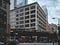# Object-Oriented JavaScript — Conditionals and LoopsPhoto by Tine Ivanič on Unsplash

JavaScript is partly an object-oriented language.

To learn JavaScript, we got to learn the object-oriented parts of JavaScript.

In this article, we’ll look at conditionals and loops.

# Ternary Operator

The ternary operator is a shorter version of the if-else syntax.

For instance, instead of writing:

`let a = 3;let result = '';if (a === 1) {  result = "a is 3";} else {  result = "a is not 3";}`

We can write:

`let a = 1;let result = (a === 3) ? "a is 3" : "a is not 3";`

The ternary expression can be added with the `?` and `:` symbols.

`a === 3` is the conditional expression.

And the strings are what we return.

# Switch

If we have lots of `if` conditions and `else...if` parts, then we can use the `switch` statements to write them,

For instance, we can write:

`let a = '1',  result = '';switch (a) {  case 1:    result = 'Number';    break;  case '1':    result = 'String';    break;  default:    result = ''    break;}`

We have a `switch` statement with `case` clauses inside.

The `case` clause value is compared against `a` and the correct clause would be run based on the match.

We need the `break` keyword so that we can stop at the end of the clause.

The `default` clause is run when none of the other cases match.

# Loops

Loops let us run code repeatedly.

JavaScript has the following loops:

• `while` loops
• `do-while` loops
• `for` loops
• `for-in` loops
• `for-of` loops

The `while` loop takes a condition and runs some code.

For instance, we can write:

`let i = 0;while (i < 10) {  i++;}`

The code runs the `while` loop which increments `i` by 1 until it reaches 10.

The parentheses surrounds the condition to run the loop.

As long as the condition is `true` , it’ll be run.

The `do...while` loop is a slight variation of the `while` loop.

It’s different from the `while` loop in that the first iteration is always run.

We can use it by writing:

`let i = 0;do {  i++;} while (i < 10);`

`i` is always incremented once.

Then the whether more iterations are run are determined by the condition inside the parentheses.

The `for` loop is the most widely used kind of loop.

It has the initialization clause which initializes the variable which we want to iterate.

The increment clause updates the variable.

The ending condition is also added so it can end.

For instance, we can write:

`for (let i = 0; i < 100; i++) {  console.log(i)}`

We keep incrementing `i` until it reaches 100.

We can vary this by moving the body to the loop heading:

`for (let i = 0; i < 100; i++, console.log(i)) {  }`

But it doesn’t make much sense to do that.

Loops can be nested within each other.

For instance, we can write:

`for (let i = 0; i < 100; i++) {  for (let j = 0; j < 100; j++) {    console.log(i, j)  }}`

We have one loop nested in another loop.Photo by Johannes Krupinski on Unsplash

# Conclusion

The ternary operator lets us write `if...else` statements in a shorter way.

And we can create loops in various ways.

Web developer. Subscribe to my email list now at https://thewebdev.info/subscribe/. Email me at hohanga@gmail.com

Web developer. Subscribe to my email list now at https://thewebdev.info/subscribe/. Email me at hohanga@gmail.com